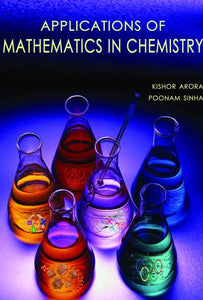Applications of Mathematics in Chemistry

Vendor

MJP PUBLISHERS

Type

Books

Categories
100 in stock.
Quantity must be 1 or more
Regular price Rs. 1,200.00

Introduction to Vectors
Applications of Vectors in Chemistry
Matrix Algebra
Applications of Matrices in Chemistry
Differential Calculus
Applications of Differential Calculus in Chemistry
Integral Calculus
Applications of Integral Calculus in Chemistry
Differential Equations in Chemistry
Permutation, Combination and Probability
Applications of Permutation, Combination and Probability in Chemistry
Statistics
Applications of Statistics in Chemistry
Sale

Unavailable

Sold Out